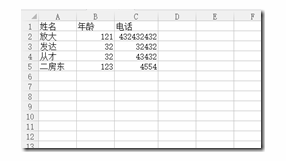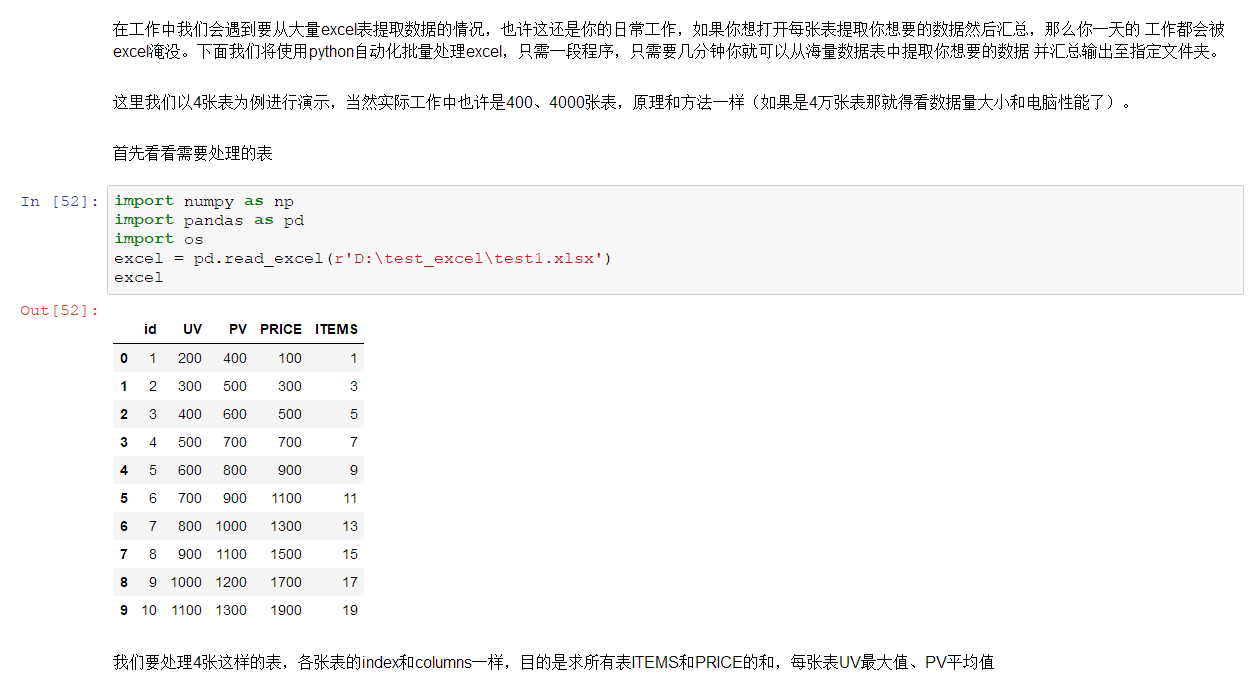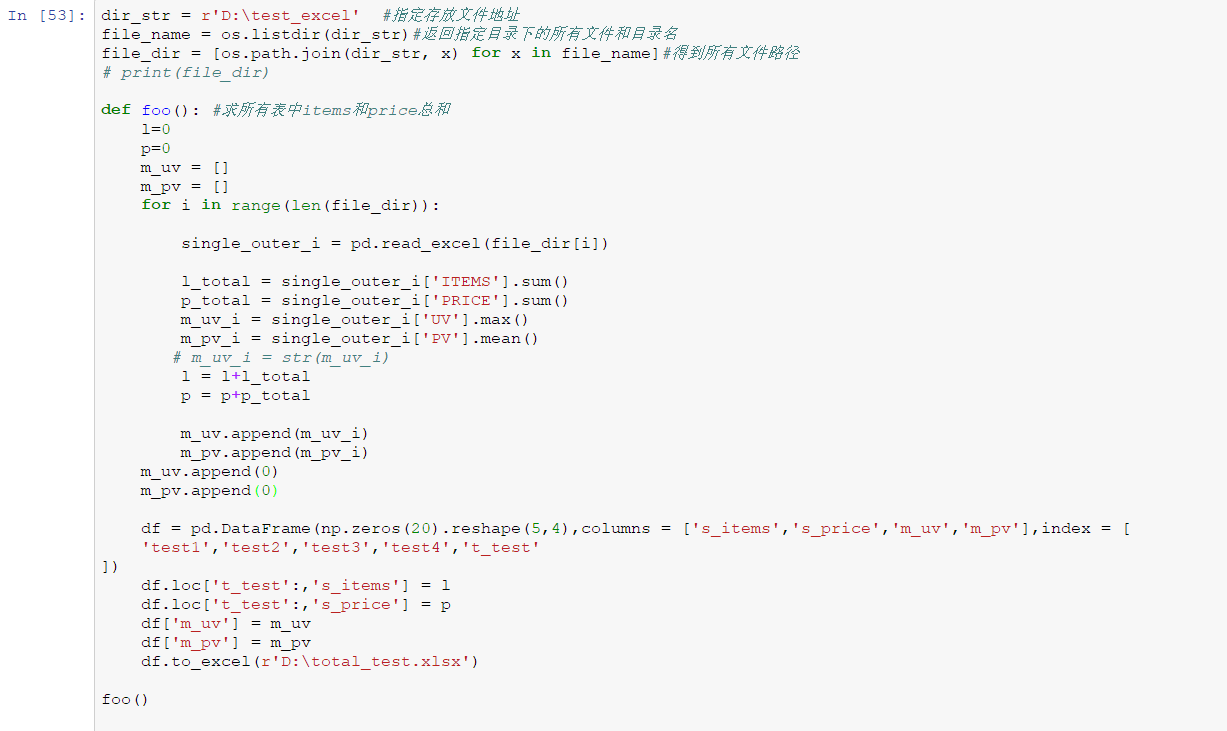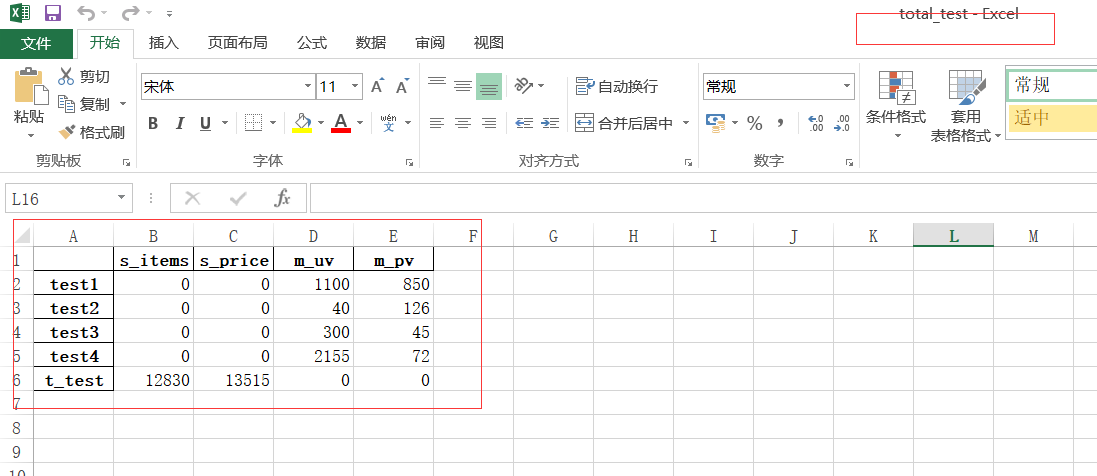• 1.通过实战处理Excel表格学习Python基础 2.通过学习Python基础快速处理Excel数据 3.快速掌握Python基础语法及用法Excel Python基础 编程语言 Python实战
• 包括4个pdf教程：python处理excel的教程、利用pandas处理Excel数据、Python进阶： Excel 基本操作、Python办公自动化之Excel做表自动化
• ## python高手之路python处理excel文件

万次阅读 多人点赞 2020-03-29 18:57:31
python处理excel文件主要是第三方模块库xlrd、xlwt、xluntils和pyExcelerator，除此之外，python处理excel还可以用win32com和openpyxl模块。 方法一 小罗问我怎么从excel中读取数据，然后我百了一番，做下记录...
用python来自动生成excel数据文件。python处理excel文件主要是第三方模块库xlrd、xlwt、xluntils和pyExcelerator，除此之外，python处理excel还可以用win32com和openpyxl模块。

方法一 小罗问我怎么从excel中读取数据，然后我百了一番，做下记录excel数据图(小罗说数据要给客户保密，我随手写了几行数据)：#!/usr/bin/env python
# -*- coding: utf-8 -*-
# 读取excel数据
# 小罗的需求，取第二行以下的数据，然后取每行前13列的数据
import xlrd
data = xlrd.open_workbook('test.xls') # 打开xls文件
table = data.sheets() # 打开第一张表
nrows = table.nrows 	 # 获取表的行数
for i in range(nrows): 	 # 循环逐行打印
if i == 0: # 跳过第一行
continue
print table.row_values(i)[:13] # 取前十三列

excel的写操作等后面用到的时候在做记录
方法二 使用xlrd读取文件，使用xlwt生成Excel文件（可以控制Excel中单元格的格式）。但是用xlrd读取excel是不能对其进行操作的；而xlwt生成excel文件是不能在已有的excel文件基础上进行修改的，如需要修改文件就要使用xluntils模块。pyExcelerator模块与xlwt类似，也可以用来生成excel文件。
**1.[代码]test_xlrd.py **
#coding=utf-8
#######################################################
#filename:test_xlrd.py
#author:defias
#date:xxxx-xx-xx
#function：读excel文件中的数据
#######################################################
import xlrd
#打开一个workbook
workbook = xlrd.open_workbook('E:\\Code\\Python\\testdata.xls')
#抓取所有sheet页的名称
worksheets = workbook.sheet_names()
print('worksheets is %s' %worksheets)
#定位到sheet1
worksheet1 = workbook.sheet_by_name(u'Sheet1')
"""
#通过索引顺序获取
worksheet1 = workbook.sheets()
#或
worksheet1 = workbook.sheet_by_index(0)
"""
"""
#遍历所有sheet对象
for worksheet_name in worksheets:
worksheet = workbook.sheet_by_name(worksheet_name)
"""
#遍历sheet1中所有行row
num_rows = worksheet1.nrows
for curr_row in range(num_rows):
row = worksheet1.row_values(curr_row)
print('row%s is %s' %(curr_row,row))
#遍历sheet1中所有列col
num_cols = worksheet1.ncols
for curr_col in range(num_cols):
col = worksheet1.col_values(curr_col)
print('col%s is %s' %(curr_col,col))
#遍历sheet1中所有单元格cell
for rown in range(num_rows):
for coln in range(num_cols):
cell = worksheet1.cell_value(rown,coln)
print cell
"""
#其他写法：
cell = worksheet1.cell(rown,coln).value
print cell
#或
cell = worksheet1.row(rown)[coln].value
print cell
#或
cell = worksheet1.col(coln)[rown].value
print cell
#获取单元格中值的类型，类型 0 empty,1 string, 2 number, 3 date, 4 boolean, 5 error
cell_type = worksheet1.cell_type(rown,coln)
print cell_type
"""

**2. [代码]test_xlwt.py **
#coding=utf-8
#######################################################
#filename:test_xlwt.py
#author:defias
#date:xxxx-xx-xx
#function：新建excel文件并写入数据
#######################################################
import xlwt
#创建workbook和sheet对象
workbook = xlwt.Workbook() #注意Workbook的开头W要大写
#向sheet页中写入数据
sheet1.write(0,0,'this should overwrite1')
sheet1.write(0,1,'aaaaaaaaaaaa')
sheet2.write(0,0,'this should overwrite2')
sheet2.write(1,2,'bbbbbbbbbbbbb')
"""
#-----------使用样式-----------------------------------
#初始化样式
style = xlwt.XFStyle()
#为样式创建字体
font = xlwt.Font()
font.name = 'Times New Roman'
font.bold = True
#设置样式的字体
style.font = font
#使用样式
sheet.write(0,1,'some bold Times text',style)
"""
#保存该excel文件,有同名文件时直接覆盖
workbook.save('E:\\Code\\Python\\test2.xls')
print '创建excel文件完成！'


**3. [代码]test_xlutils.py **
#coding=utf-8
#######################################################
#filename:test_xlutils.py
#author:defias
#date:xxxx-xx-xx
#function：向excel文件中写入数据
#######################################################
import xlrd
import xlutils.copy
#打开一个workbook
rb = xlrd.open_workbook('E:\\Code\\Python\\test1.xls')
wb = xlutils.copy.copy(rb)
#获取sheet对象，通过sheet_by_index()获取的sheet对象没有write()方法
ws = wb.get_sheet(0)
#写入数据
ws.write(1, 1, 'changed!')
#添加sheet页
#利用保存时同名覆盖达到修改excel文件的目的,注意未被修改的内容保持不变
wb.save('E:\\Code\\Python\\test1.xls')


#coding=utf-8
#######################################################
#author:defias
#date:xxxx-xx-xx
#function：读excel文件中的数据
#######################################################
import pyExcelerator
#parse_xls返回一个列表，每项都是一个sheet页的数据。
#每项是一个二元组(表名,单元格数据)。其中单元格数据为一个字典，键值就是单元格的索引(i,j)。如果某个单元格无数据，那么就不存在这个值
sheets = pyExcelerator.parse_xls('E:\\Code\\Python\\testdata.xls')
print sheets


**5. [代码]test_pyExcelerator.py **
#coding=utf-8
#######################################################
#filename:test_pyExcelerator.py
#author:defias
#date:xxxx-xx-xx
#function：新建excel文件并写入数据
#######################################################
import pyExcelerator
#创建workbook和sheet对象
wb = pyExcelerator.Workbook()
#设置样式
myfont = pyExcelerator.Font()
myfont.name = u'Times New Roman'
myfont.bold = True
mystyle = pyExcelerator.XFStyle()
mystyle.font = myfont
#写入数据，使用样式
ws.write(0,0,u'ni hao 帕索！',mystyle)
#保存该excel文件,有同名文件时直接覆盖
wb.save('E:\\Code\\Python\\mini.xls')
print '创建excel文件完成！'


展开全文df1.ix[1:3,['merge_add']] #取某一列的前3行
excel的内容需要有规律，python才能按顺序读取出来。 比如按行按列读取。
一、安装xlrd模块
到python官网下载http://pypi.python.org/pypi/xlrd模块安装，前提是已经安装了python 环境。
二、使用介绍
1、导入模块
import xlrd
2、打开Excel文件读取数据
data = xlrd.open_workbook('excelFile.xls')
3、使用技巧
获取一个工作表
table = data.sheets() #通过索引顺序获取
table = data.sheet_by_index(0) #通过索引顺序获取
table = data.sheet_by_name(u'Sheet1')#通过名称获取
获取整行和整列的值（数组）
table.row_values(i)
table.col_values(i)
获取行数和列数
nrows = table.nrows
ncols = table.ncols
循环行列表数据
for i in range(nrows ):
print table.row_values(i)
单元格
cell_A1 = table.cell(0,0).value
cell_C4 = table.cell(2,3).value
使用行列索引
cell_A1 = table.row(0).value
cell_A2 = table.col(1).value
简单的写入
row = 0
col = 0
# 类型 0 empty,1 string, 2 number, 3 date, 4 boolean, 5 error
ctype = 1 value = '单元格的值'
xf = 0 # 扩展的格式化
table.put_cell(row, col, ctype, value, xf)
table.cell(0,0) #单元格的值'
table.cell(0,0).value #单元格的值'
展开全文• 资源包含使用python读取excel文档，创建excel文档，和修改excel文档，代码注释清晰，适合初学者入门
• python处理excel的常见使用 基本的excel处理方法
• ## 使用python批量处理excel

万次阅读 多人点赞 2018-03-24 18:02:50
看看结果： 完整代码扫描下方二维码回复【pyexcel】获取。。： 有酒有风看看结果：完整代码扫描下方二维码回复【pyexcel】获取。。：

有酒有风


展开全文• 1.快速自动化处理重复表格数据 2.快速掌握openpyxl库 3.实战操作处理海量数据 4.加深Python学习Python基础教程 Python基础
• 主要介绍了Python读写处理Excel表格，文中通过示例代码介绍的非常详细，对大家的学习或者工作具有一定的参考学习价值，需要的朋友们下面随着小编来一起学习学习吧
• ## python处理中文Excel

万次阅读 2014-05-09 17:05:06
如果Python处理Excel，需要一个
如果Python要处理Excel，需要一个“xlrd"包, [ xls read], download line: http://pypi.python.org/pypi/xlrd
cd 到目录下边：
输入：python setup.py install 安装即可
读取很简单，网上随便一搜都会有，例如按行读取:

import xlrd
#import chardet
data = xlrd.open_workbook("emotion.xlsx")
table = data.sheets()
nrows = table.nrows
for i in range(nrows):
#print chardet.detect(str(table.row_values(i)))
print table.row_values(i)

可是这样在Linux Terminal 打印出来的是乱码，把打印语句改成：
 	print str(table.row_values(i)).decode("unicode_escape").encode("utf8")

为什么是这样？ 可以从Ubuntu 的系统设置中看到，控制器显示的字符是UTF8的，所以最后需要encode成UTF8的，2.为什么要用decode? 因为要encode成UTF8，得是unicode格式的字符串才行，但是默认的字符串是str型的，所有需要把其他的字符编码转成UNICODE才行，然后，因为打印出来的乱码是. u'\XXX' 这种形式， 所以根据经验应该是unicode_escape形式。 okay，说完啦。


展开全文linux ubuntu
• pythonexcel数据处理可视化的方法：首先安装xlrd与xlwt库，进行表格读取；然后使用pyecharts生成Echarts图表的类库；最后安装Echarts读取Excel数据及显示即可。pythonexcel数据处理可视化的方法：Excel表操作...
• 用python来自动生成excel数据文件。python处理excel文件主要是第三方模块库xlrd、xlwt、xluntils和pyExcelerator，除此之外，python处理excel还可以用win32com和openpyxl模块
• ## 零基础使用Python读写处理Excel表格

万次阅读 多人点赞 2016-12-22 14:17:04
零基础实例详细讲解如何使用Python第三方库处理Excel表格解放劳动力
• python3 处理Excel 常见问题-读取Excel中时间 https://blog.csdn.net/fenghuajianshi/article/details/78263092时间
• 刀位点信息.png 利用python处理excel文件的数据，通过利用python处理由excel转化来的txt文件包含的数据
• 本篇文章主要介绍了Python处理Excel文件实例代码，小编觉得挺不错的，现在分享给大家，也给大家做个参考。一起跟随小编过来看看吧
• 引由于需要解决大批量Excel处理的事情，与其手工操作还不如写个简单的代码来处理，大致选了一下感觉还是Python最容易操作。安装库Python环境首先当然是配环境，不过选Python的一个重要原因就是Mac内是自带Python环境...
• 最近由于比赛需求，需要大量的数据支持系统的功能测试。然后比较坑的是，所有的源数据都是来自Excel文件格式。... 于是就想到了我们伟大的python，可以说python这种语言用来处理批量数据实在是再好...Pandas
• 批量删除多个Excel文件中的同一指定行 场景：在文件夹数据资料中，有多个Excel文件，每个Excel文件的格式相同，如下图所示： 现在需要删除每个Excel文件中的指定行，如下图所示，要删除Excel文件中某个Sheet的第...win32com openpyxl...

# python能处理excel吗python 订阅